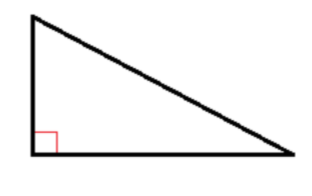# Different Types of Triangles

August 16, 2020
SAC TutoringThere are many types of triangles that you will come across in math. In this post we are going to look at the 6 most common triangles you will see in math questions.

1. A triangle consists of 3 sides.
2. The sum of all angles in a triangle is 180°

These two rules are true for ANY type of triangle. Keep this in mind as we go through the different types.

## 1. Right angle triangle

The little square in the corner means the angle equals 90°

## 2. Acute triangle

This is where all corners of triangles are acute angles (smaller than 90°)

## 3. Obtuse triangle

This triangle has one corner that contains a obtuse angle (larger than 90°)

## 4. Equilateral triangle

When all sides and angles are the same and all of them equal 60°

## 5. Isosceles triangle

Two of the sides and two of the angles are the same.

## 6. Scalene triangle

No sides or angles are equal to one another.

You will use these triangles in math all throughout high school so make sure you are familiar with all the different triangles!

If you want more information or more personalized help, check out the rest of our website and enroll in a trial class now!

Study smarter, not harder, with SAC.